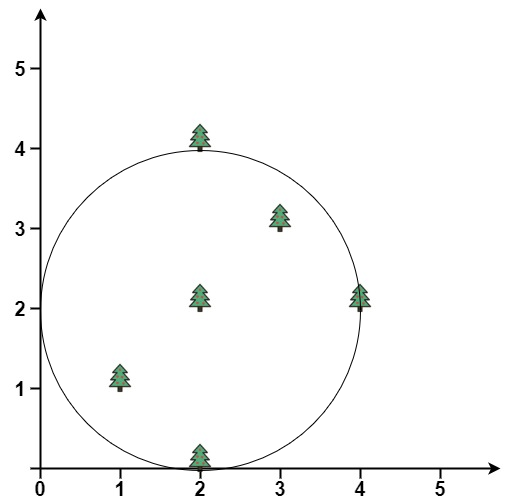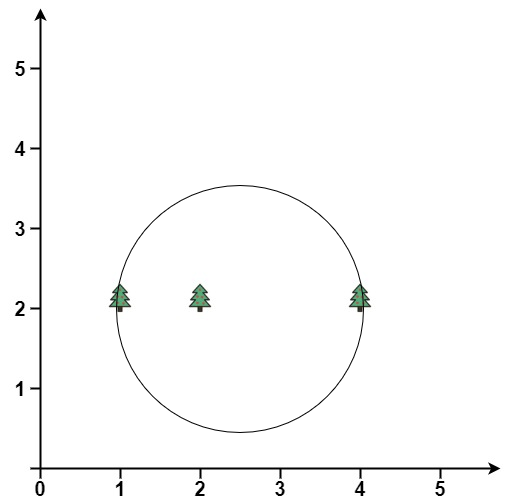Formatted question description: https://leetcode.ca/all/1924.html

# 1924. Erect the Fence II

Hard

## Description

You are given a 2D integer array trees where trees[i] = [x_i, y_i] represents the location of the i-th tree in the garden.

You are asked to fence the entire garden using the minimum length of rope possible. The garden is well-fenced only if all the trees are enclosed and the rope used forms a perfect circle. A tree is considered enclosed if it is inside or on the border of the circle.

More formally, you must form a circle using the rope with a center (x, y) and radius r where all trees lie inside or on the circle and r is minimum.

Return the center and radius of the circle as a length 3 array [x, y, r]. Answers within 10-5 of the actual answer will be accepted.

Example 1:Input: trees = [[1,1],[2,2],[2,0],[2,4],[3,3],[4,2]]

Output: [2.00000,2.00000,2.00000]

Explanation: The fence will have center = (2, 2) and radius = 2

Example 2:Input: trees = [[1,2],[2,2],[4,2]]

Output: [2.50000,2.00000,1.50000]

Explanation: The fence will have center = (2.5, 2) and radius = 1.5

Constraints:

• 1 <= trees.length <= 3000
• trees[i].length == 2
• 0 <= x_i, y_i <= 3000

## Solution

This problem is the smallest-circle problem. Use Welzl’s algorithm to find the smallest circle’s center and radius.

class Solution {
public double[] outerTrees(int[][] trees) {
double radius = 0.0;
double x = trees, y = trees;
int length = trees.length;
for (int i = 1; i < length; i++) {
if (getSquaredDistance(trees[i], x, trees[i], y) > radius * radius) {
x = trees[i];
y = trees[i];
for (int j = 0; j < i; j++) {
if (getSquaredDistance(trees[j], x, trees[j], y) > radius * radius) {
x = (trees[i] + trees[j]) / 2.0;
y = (trees[i] + trees[j]) / 2.0;
radius = Math.sqrt(getSquaredDistance(x, trees[j], y, trees[j]));
for (int k = 0; k < j; k++) {
if (getSquaredDistance(trees[k], x, trees[k], y) > radius * radius) {
double[] circle = getCircle(trees, i, j, k);
x = circle;
y = circle;
}
}
}
}
}
}
return new double[]{x, y, radius};
}

public double[] getCircle(int[][] trees, int i, int j, int k) {
double p1 = trees[i] - trees[k], p2 = trees[i] - trees[j];
double q1 = trees[i] - trees[k], q2 = trees[i] - trees[j];
double a = (trees[i] * trees[i] - trees[j] * trees[j]) + (trees[i] * trees[i] - trees[j] * trees[j]);
double b = (trees[i] * trees[i] - trees[k] * trees[k]) + (trees[i] * trees[i] - trees[k] * trees[k]);
double c = 2 * (trees[i] - trees[j]) * (trees[i] - trees[k]) - 2 * (trees[i] - trees[k]) * (trees[i] - trees[j]);
double x = (p1 * a - p2 * b) / c;
double y = (q2 * b - q1 * a) / c;
double radius = Math.sqrt(getSquaredDistance(trees[k], x, trees[k], y));
return new double[]{x, y, radius};
}

public double getSquaredDistance(double x1, double x2, double y1, double y2) {
return (x1 - x2) * (x1 - x2) + (y1 - y2) * (y1 - y2);
}
}